# Swift 与谷歌的可微编程项目## 谷歌接受了挑战

• Go ：他们在文档中指出，Go 过于依赖其接口提供的动态调度，为了实现想要的功能，他们不得不对语言进行重大修改。这违背了 Go 提倡的简单和体积小的哲学。相反，Swift 的协议和扩展提供了很多自由，你可以根据需要定义调度的静态程度。而且，Swift 语言已经非常复杂了（而且在变得越来越复杂），因此，让它更复杂一点以适应谷歌想要的特性并不会造成很大的问题。
• C++ &  Rust ：谷歌的目标用户群是那些大部分时间都在使用 Python 的人，他们更感兴趣的是花时间考虑模型和数据，而不是管理内存分配或所有权之类的事情。Rust 和 C++ 具有一定的复杂性和对底层细节的关注，这在进行数据科学 / 机器学习开发时通常是不合理的。
• Julia ：如果你在 HackerNews Reddit 上阅读任何有关 S4TF 的帖子，第一个评论通常是“为什么他们不选择 Julia？”谷歌在档中提到，Julia 看起来也很有前途，但是，至于就为什么没有选择它的原因并没有提供坚实的理由。文中提到，Swift 社区比 Julia 大得多，这是事实，但 Julia 科学和数据科学社区比 Swift 大得多，这些社区使用 S4TF 可能会更多。需要记住的是，谷歌团队在 Swift 方面更专业，因为 Swift 的创始人 Chris Lattner 启动了这个项目，所以这可能在决定中起了很大的作用。
• 一门新语言：我认为他们在宣言中说得很好：“创造一门语言是一项工作量大得荒谬的工作”。这将花费太长时间，而且很可能跟不上机器学习的发展速度。

## Swift 酷在哪？

### 1. 速度快

Swift 很快。这是我开始使用 Swift 时首先测试的。我写了几个简短的脚本，并将它们与 Python 和 C 进行比较。说实话，这些测试并不很复杂，只是用整数填充一个数组，然后把它们都加起来。我承认，这种方法不足以全面测试 Swift 的真实速度，但更让我好奇的是，就算 Swift 没法在速度上与 C 全面看齐，至少能在某些场景下追上 C 的速度。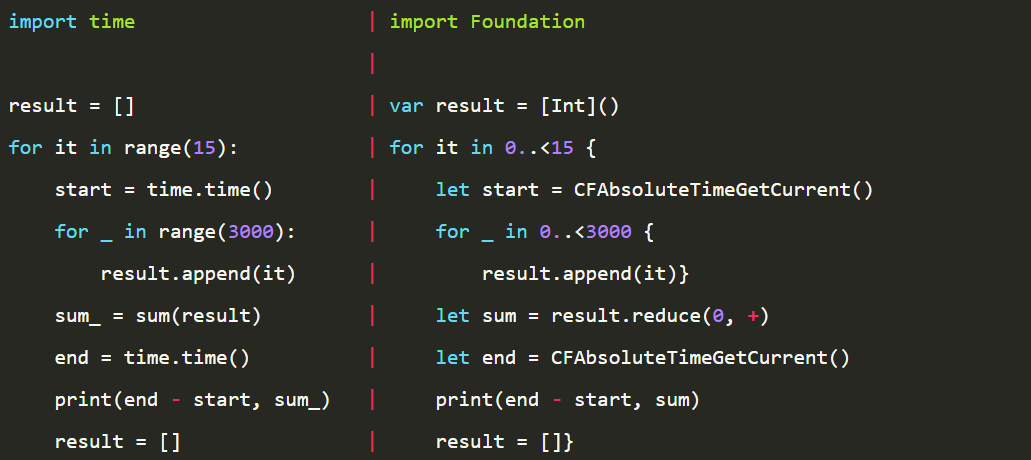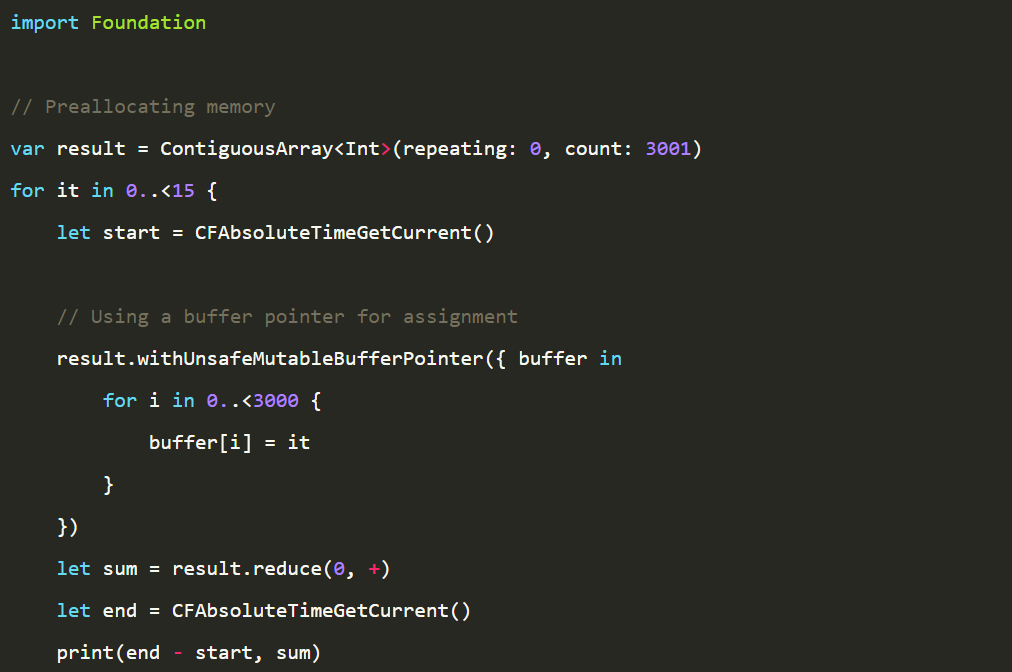### 2. 函数签名巧妙

Swift 的函数签名采取了一种有趣的方式，其最基本的形式相对比较简单：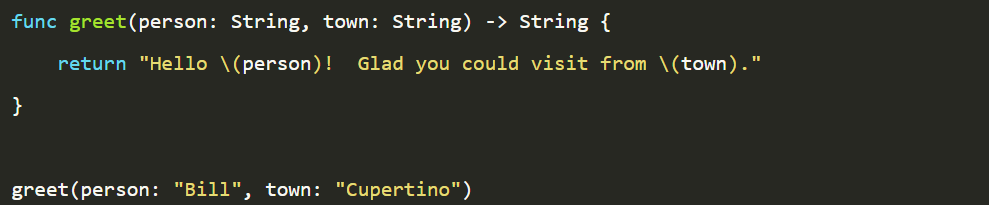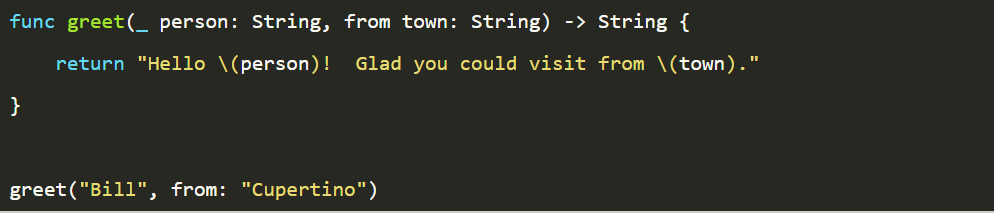### 3. 大量使用闭包

Swift 大量使用了闭包。因此，它有一些快捷方式，使其更加人性化。这个例子来自该语言的文档，着重说明了这些快捷方式如何使 Swift 简洁而富有表现力。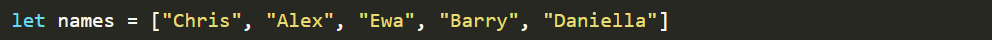)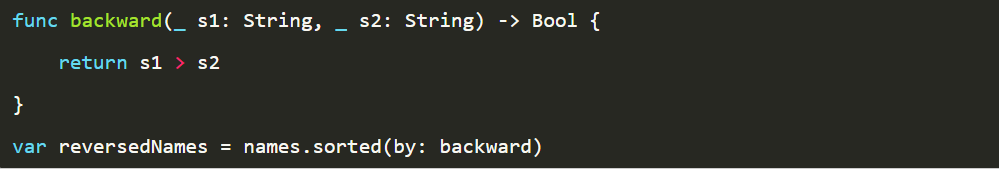backward 函数一次比较两个数据项，如果它们的顺序符合要求，则返回 true；如果不符合要求，则返回 false。数组的 sorted 方法需要这样一个函数作为输入才能知道如何对数组进行排序。顺便说一下，我们还可以看到，这里使用了参数标签，非常简洁。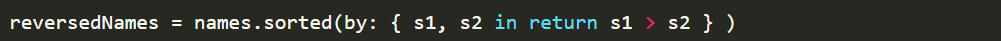{}之间的代码是定义一个闭包，同时将其作为一个参数传递。如果你从未听说过闭包，那么我稍微说明下，闭包是捕获其上下文的未命名函数。我们可以把它们看做是加强型的 Python lambdas。闭包中的关键字 in 用于分隔闭包的参数及其主体。更直观的关键字，如: 已经被签名类型定义占用（闭包的参数类型可以从 sorted 的方法签名自动推断，这种情况是可以避免的）在编程中，命名一个东西是最困难的事情之一，我们坚持使用不那么直观的 in 关键字。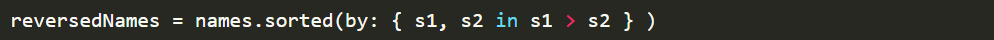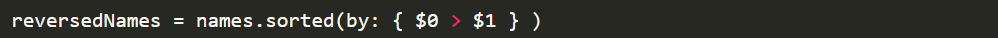Swift 还隐式地命名了位置参数，所以在上面的例子中，$0 是第一个参数，$1 是第二个参数，\$2 是第三个参数，依此类推。目前代码已经很紧凑了，而且很容易理解，但我们可以更进一步：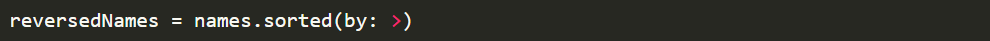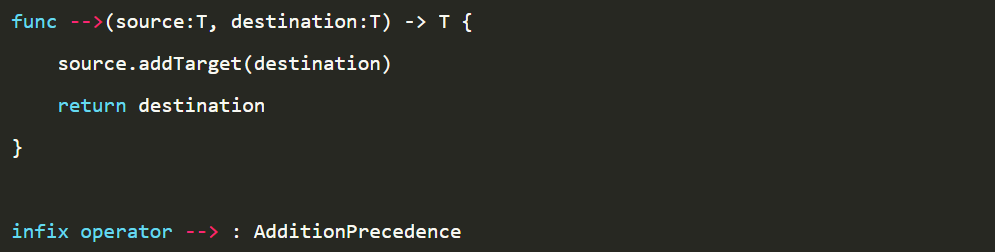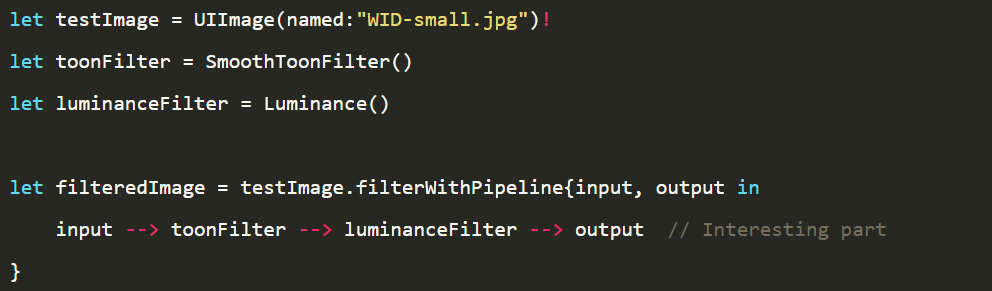### 4. 基本类型是在标准库中定义的结构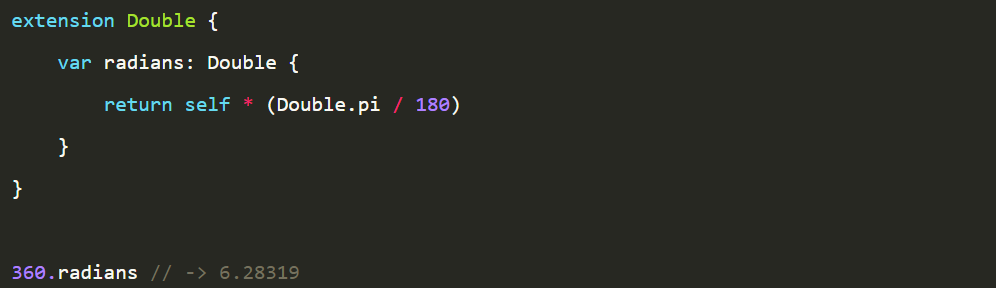## 可微编程

### 一门可微语言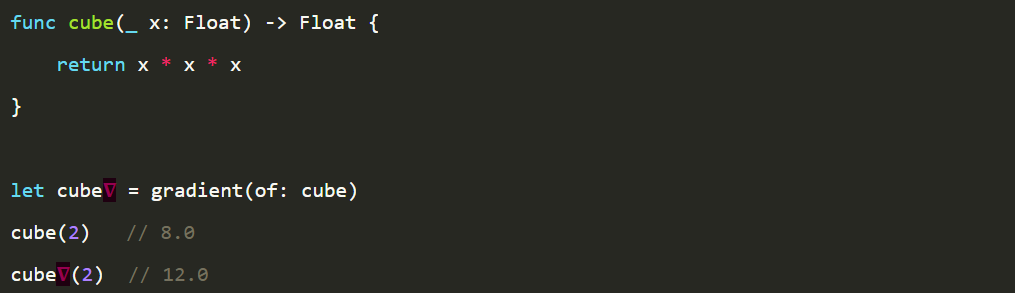struct Perceptron: @memberwise Differentiable {    var weight: SIMD2<Float> = .random(in: -1..<1)    var bias: Float = 0    @differentiable    func callAsFunction(_ input: SIMD2<Float>) -> Float {        (weight * input).sum() + bias    }}var model = Perceptron()let andGateData: [(x: SIMD2<Float>， y: Float)] = [    (x: [0， 0]， y: 0)，    (x: [0， 1]， y: 0)，    (x: [1， 0]， y: 0)，    (x: [1， 1]， y: 1)，]for _ in 0..<100 {    let (loss， 𝛁loss) = valueWithGradient(at: model) { model -> Float in        var loss: Float = 0        for (x， y) in andGateData {            let ŷ = model(x)            let error = y - ŷ            loss = loss + error * error / 2        }        return loss    }    print(loss)    model.weight -= 𝛁loss.weight * 0.02    model.bias -= 𝛁loss.bias * 0.02}

### 微分外部代码

import Glibc  // we import pow and log from herefunc powerOf2(_ x: Float) -> Float {    return pow(2， x)}@derivative(of: powerOf2)func dPowerOf2d(_ x: Float) -> (value: Float， pullback: (Float) -> Float) {    let d = powerOf2(x) * log(2)    return (value: d， pullback: { v in v * d })}powerOf2(3)，               // 8gradient(of: powerOf2)(3)  // 5.545

Glibc 是一个 C 语言库，所以 Swift 编译器不知道其操作的导数是什么。我们可以使用 @derivative 给编译器提供这些信息，然后就可以轻松地使用这些外部操作和本地操作形成一个大的可微网络。在本例中，我们从 Glibc 导入 pow 和 log，并使用它们创建函数 powerOf2 及其导函数。

TensorFlow Library for Swift 的当前版本就是使用这个特性构建的。该库从 TF Eager 库的 C API 中导入所有的操作，但是它没有插入 TensorFlow 的自动微分系统，而是指定了每个基本操作的导数，并让Swift 来处理它。然而，并不是所有的操作都需要，因为许多操作是更基本操作的组合，因此，Swift 可以自动推断出它们的导数。然而，基于TF Eager 库的当前版本有一个很大的缺点：TF Eager 确实很慢，因此，Swift 版本也很慢。这似乎只是一个暂时的问题，它会随着 XLA （通过 x10）和 MLIR 的合并而得到修复。

import TensorFlowlet hiddenSize: Int = 10struct IrisModel: Layer {    var layer1 = Dense<Float>(inputSize: 4， outputSize: hiddenSize， activation: relu)    var layer2 = Dense<Float>(inputSize: hiddenSize， outputSize: hiddenSize， activation: relu)    var layer3 = Dense<Float>(inputSize: hiddenSize， outputSize: 3)    @differentiable    func callAsFunction(_ input: Tensor<Float>) -> Tensor<Float> {        return input.sequenced(through: layer1， layer2， layer3)    }}var model = IrisModel()let optimizer = SGD(for: model， learningRate: 0.01)let (loss， grads) = valueWithGradient(at: model) { model -> Tensor<Float> in    let logits = model(firstTrainFeatures)    return softmaxCrossEntropy(logits: logits， labels: firstTrainLabels)}print("Current loss: \(loss)")

### Python 互操作

import Pythonprint(Python.version)let np = Python.import("numpy")let plt = Python.import("matplotlib.pyplot")// let time = np.arange(0， 10， 0.01)let time = Array(stride(from: 0， through: 10， by: 0.01)).makeNumpyArray()let amplitude = np.exp(-0.1 * time)let position = amplitude * np.sin(3 * time)plt.figure(figsize: [15， 10])plt.plot(time， position)plt.plot(time， amplitude)plt.plot(time， -amplitude)plt.xlabel("Time (s)")plt.ylabel("Position (m)")plt.title("Oscillations")plt.show()

## 结论

Swift: Google’s bet on differentiable programming